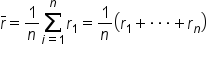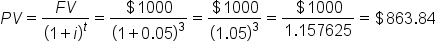+
Present Value, Single Cash Flows
Time Value of Money and Financial Securities
0
of 0 possible points

# Present Value, Single Cash Flows

Author: Sophia Tutorial
##### Description:

Calculate the present value of a single-period or multi-period investment.

(more)### Developing Effective Teams

*No strings attached. This college course is 100% free and is worth 1 semester credit.

37 Sophia partners guarantee credit transfer.

299 Institutions have accepted or given pre-approval for credit transfer.

* The American Council on Education's College Credit Recommendation Service (ACE Credit®) has evaluated and recommended college credit for 32 of Sophia’s online courses. Many different colleges and universities consider ACE CREDIT recommendations in determining the applicability to their course and degree programs.

Tutorial

what's covered
In this lesson, you will learn how to calculate the present value of a single-period and multi-period investment over time. Specifically, this lesson will cover:
1. The Discount Rate
2. Formulas
1. Single-Period Investment
2. Multi-Period Investment
3. Calculating Present Value

## 1. The Discount Rate

The time value of money framework says that money in the future is not worth as much as money in the present. Investors would prefer to have the money today because then they are able to spend it, save it, or invest it right now instead of having to wait to be able to use it.

The difference between what the money is worth today and what it will be worth at a point in the future can be quantified. The value of the money today is called the present value (PV), and the value of the money in the future is called the future value (FV).

Another common term for finding present value (PV) is discounting . Discounting is the procedure of finding what a future sum of money is worth today. As you know from the previous sections, to find the PV of a payment, you need to know the future value (FV), the number of time periods in question, and the interest rate. The interest rate, in this context, is more commonly called the discount rate.

The discount rate is the term for the cost of not having the money today.

EXAMPLE

If the interest rate is 3% per year, it means that you would be willing to pay 3% of the money to have it one year sooner.

It also represents some cost (or group of costs) to the investor or creditor. The sum of these costs amounts to a percentage which becomes the interest rate (plus a small profit, sometimes).

Here are some of the most significant costs from the investor/creditor’s point of view:

• Opportunity Cost: This is the cost of not having the cash on hand at a certain point of time. If the investor/creditor had the cash, they could spend it. But since it has been invested/loaned out, they incur the cost of not being able to spend it.
• Inflation: The real value of a single dollar decreases over time with inflation. That means that even if everything else is constant, a \$100 item will retail for more than \$100 in the future. Inflation is generally positive in most countries at most times (if it’s not, it’s called deflation, but it’s rare).
• Risk: There is a chance that you will not get your money back because it is a bad investment, and the debtor defaults. You require compensation for taking on that risk.
• Liquidity: Investing or loaning out cash necessarily reduces your liquidity.
All of these costs combine to determine the interest rate on an account, and that interest rate, in turn, is the rate at which the sum is discounted.

terms to know

Discounting
The process of finding the present value using the discount rate.
Discount Rate
The interest rate used to discount future cash flows of a financial instrument; the annual interest rate used to decrease the amounts of future cash flow to yield their present value.

## 2. Formulas

The PV and the discount rate are related through the same formula we have been using for a future value of a single payment. The equation can be rearranged to solve for PV of a single amount of money. That is, it tells you what a single payment is worth today, but not what a series of payments is worth today (that will come later). In order to find the PV, you must know the FV, i, and n.

big idea
If FV and n are held as constants, then as the discount rate (i) increases, PV decreases. PV and the discount rate, therefore, vary inversely, a fundamental relationship in finance.

2a. Single-Period Investment
With single-period investments, the concept of the time value of money is relatively straightforward. The future value is simply the present value applied to the interest rate compounded one time. So, by definition, the variable for time, n, is equal to one. If we update the formula with this information, and rearrange it so we are solving for PV, this simply means that the PV is FV divided by 1+i.

formula

Single-Period InterestThere is a cost to not having the money for one year, which is what the interest rate represents. Therefore, the PV is i% less than the FV.

2b. Multi-Period Investment
When investing, the time value of money is a core concept investors simply cannot ignore. A dollar today is valued higher than a dollar tomorrow, and when utilizing the capital, it is important to recognize the opportunity cost involved in what it could have been invested in instead.

With multi-periods in mind, interest begins to compound. Compound interest simply means that the interest from the first period is added to the future present value, and the interest rate the next time around is now being applied to a larger amount. This turns into an exponential calculation of interest, calculated as follows:

formula

Compound InterestThis means that the interest rate of a given period may not be the same percentage as the interest rate over multiple periods (in most situations). A useful tool at this point is a way to create an average rate of return over the life of the investment, which can be derived with the following:

formula

Average Rate of Returnbig idea
All and all, the difference from a time value of money perspective between single- and multiple-period investments is relatively straightforward. Normalizing expected returns in present value terms (or projecting future returns over multiple time periods of compounding interest) paints a clearer and more accurate picture of the actual worth of a given investment opportunity.

term to know

Compound Interest
An interest rate applied to multiple applications of interest during the lifetime of the investment.

## 3. Calculating Present Value

Finding the present value (PV) of an amount of money is finding the amount of money today that is worth the same as an amount of money in the future, given a certain interest rate.

Calculating the present value (PV) of a single amount is a matter of combining all of the different parts we have already discussed. But first, you must determine whether the type of interest is simple or compound interest. If the interest is simple interest, you plug the numbers into the simple interest formula. If it is compound interest, you can rearrange the compound interest formula to calculate the present value.

Once you know these three variables, you can plug them into the appropriate equation. If the problem doesn’t say otherwise, it’s safe to assume the interest compounds. If you happen to be using a program like Excel, the interest is compounded in the PV formula. Simple interest is fairly rare.

### IN CONTEXT

Suppose you would like to have \$1,000 dollars in an account after three year’s time. If the account earns 5% compounded interest yearly, how much would you have to deposit today?

Deciding how much to deposit today is the same as the present value. Since we know the future value (\$1,000), the timing (3 years), and the interest rate (5%), we can find the present value using the following formula:hint
One area where there is often a mistake is in defining the number of periods and the interest rate. They have to have consistent units, which may require some work. For example, interest is often listed as X% per year. The problem may talk about finding the PV 24 months before the FV, but the number of periods must be in years since the interest rate is listed per year. Therefore, n is 2. As long as the units are consistent, however, finding the PV is done by plug-and-chug.

term to know

Simple Interest
Interest paid only on the principal.
summary
In this lesson, you learned that the discount rate can be applied to the future value of an investment to determine the investment’s present value. Similar to an interest rate, it represents the cost of not having the money today. Like with future value, there are formulas for determining the current value of a single-period investment and a multi-period investment that utilize the discount rate. Calculating present value is useful for knowing what a future amount of money is equivalent to today.

Best of luck in your learning!

Source: THIS CONTENT HAS BEEN ADAPTED FROM LUMEN LEARNING'S “Present Value, Single Amount” TUTORIAL.

Terms to Know
Compound Interest

An interest rate applied to multiple applications of interest during the lifetime of the investment.

Discount Rate

The interest rate used to discount future cash flows of a financial instrument; the annual interest rate used to decrease the amounts of future cash flow to yield their present value.

Discounting

The process of finding the present value using the discount rate.

Simple Interest

Interest paid only on the principal.

Formulas to Know
Average Rate of ReturnCompound InterestSingle-Period InterestRating